# Numbers

 1 Introduction to Numbers 2 Pre-number Math 3 Number Names 4 PEMDAS 5 Number Systems 6 Ordinal Numbers 7 Consecutive Numbers 8 Integers 9 Natural Numbers 10 Whole Numbers 11 Even Numbers 12 Odd Numbers 13 Prime Numbers 14 Composite Numbers 15 Co-prime Numbers 16 Perfect Numbers 17 Fractions 18 Decimals 19 Rational Numbers 20 Irrational Numbers 21 Real Numbers 22 Complex Numbers 23 Factors 24 Multiples 25 Highest Common Factor (HCF) 26 Least Common Multiple (LCM) 27 Prime Factorization

## Introduction

Numbers form the basis of mathematics. We should befriend numbers in order to understand maths.

Numbers are of various kinds. We have a long list that includes ordinal numbers, consecutive numbers, odd numbers, even numbers, natural numbers, whole numbers, integers, real numbers, rational numbers, irrational numbers, and complex numbers.

Along with numbers, we come across the interesting world of factors and multiples. This world includes prime numbers, composite numbers, coprime numbers, perfect numbers (yes, numbers could be perfect!) HCF, LCM, and prime factorization.

Let’s get started on our journey of numbers.

You can go ahead and explore all important topics in Numbers by selecting the topics from this list below:

 Pre-number Math Number Names PEMDAS Number Systems Ordinal Numbers Consecutive Numbers Integers Natural Numbers Whole Numbers Even Numbers Odd Numbers Prime Numbers Composite Numbers Co-prime Numbers Perfect Numbers Fractions Decimals Rational Numbers Irrational Numbers Real Numbers Complex Numbers Factors Multiples Prime Factorization Least Common Multiple (LCM) Highest Common Factor (HCF) Number Line

## Pre-number Math

Building pre-number math skills is a prerequisite to understanding numbers. Pre-number skills like matching, sorting, classifying, ordering, and comparing will set the stage to build a strong number sense.

Example: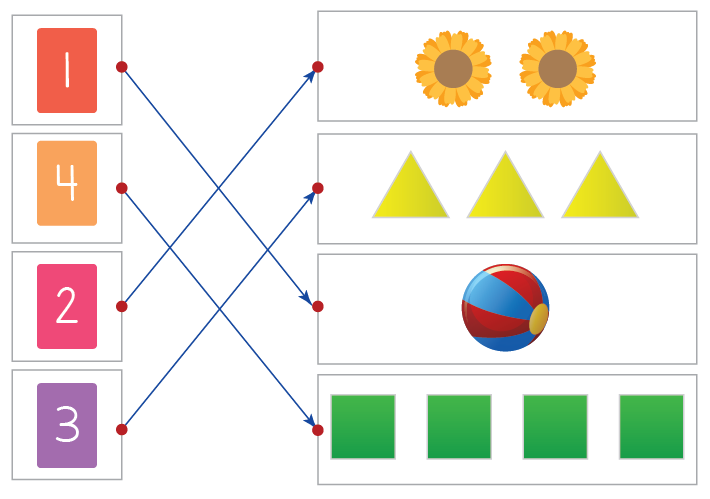In the above example, the left column displays the numbers $$1$$ to $$4.$$ The right column displays rows of items. The numbers are matched to the quantities they represent. This is an essential skill for children aged $$3$$ to $$4$$ years.

Pre-number Math skills are built-in preschool years.

Kids learn how to stand before they start taking small steps. In the same way, the pre number concept is very important for them to start understanding Mathematics. In this section, we will cover the different pre-number concepts like Matching and Sorting, Comparing and Ordering, Classification, and Shapes and patterns.

## Number Names

Number names are used to represent numbers in an alphabetical format.

Example: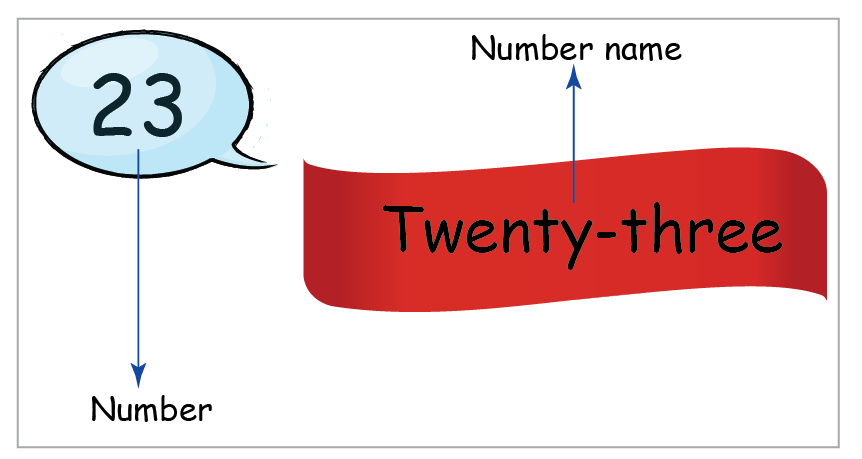## PEMDAS

The rules of PEMDAS outline the order of the operations and give structure to nested operations.

Example: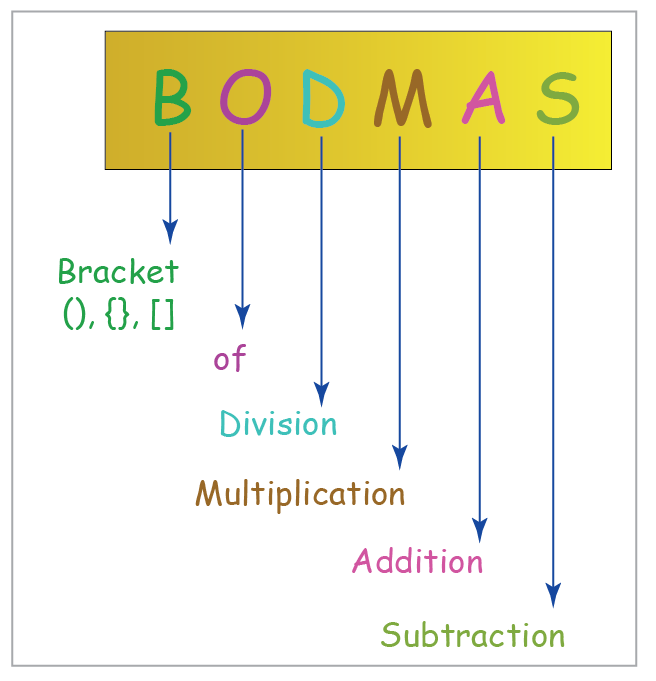We already know what PEMDAS stands for. Let’s learn in detail the different concepts of BODMAS such as Addition, Subtraction, Multiplication, and Division.

## Number Systems

The decimal number system is the most commonly used number system. The digits $$0$$ to $$9$$ are used to represent numbers. A digit in any given number has a place value.

Example: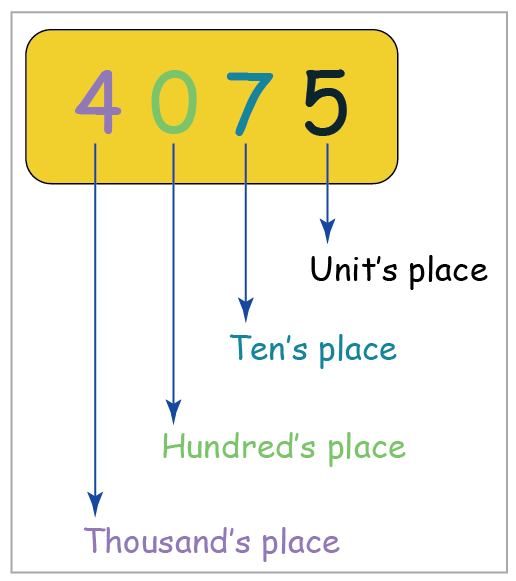The decimal number system is the standard system for denoting integers and non-integers. We will be using the decimal number system for representation of numbers up to 2-Digits, Numbers up to 3-Digits, Numbers up to 4-Digits, Numbers up to 5-Digits, Numbers up to 6-Digits, Numbers up to 7-Digits, Numbers up to 8-Digits, Numbers up to 9-Digits and Numbers up to 10-Digits.

## Ordinal Numbers

An ordinal number tells the numerical position of an object in a list. In other words, it indicates the order of things or objects, such as first, second, third, fourth, and so on.

Example: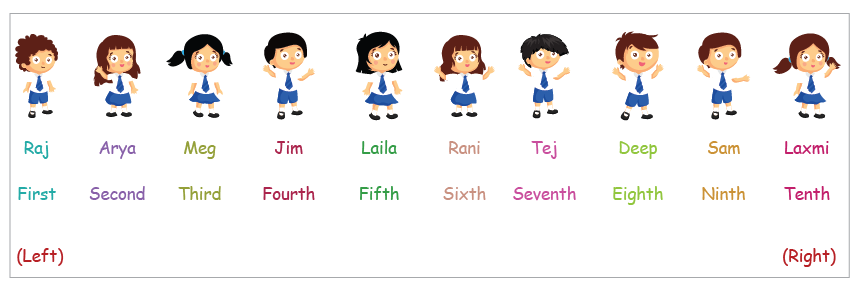In the example given above, ordinal numbers will help define the position of the children. Such as, Jim is the fourth child from the left.

## Consecutive Numbers

Consecutive numbers are numbers that follow each other in order from the smallest number to the largest number. They usually have a difference of $$1$$ between every two numbers.

Example: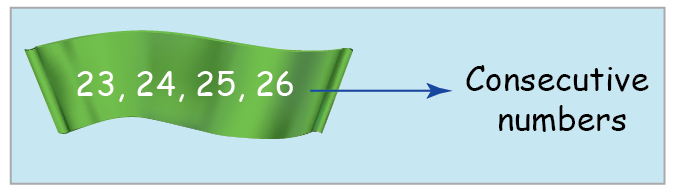## Integers

Integers are numbers that are whole numbers and negative numbers. All integers are represented by the alphabet Z.

Example: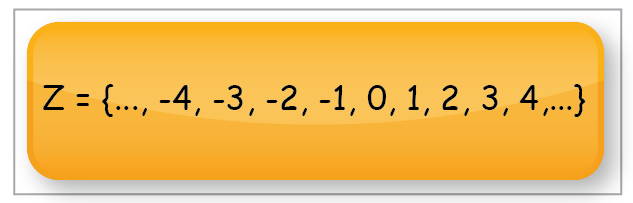A number line is full of integers. On the left side, you can find negative integers while on the right side you have the positive ones. Don’t forget the zero in between! Integers do sound interesting right? We will now learn about the Addition and Subtraction of Integers, Multiplication and Division of Integers, Euclid's Division Lemma, and Euclid's Division Algorithm.

## Natural Numbers

A natural number is a non-negative integer and is always greater than zero. It is represented by the symbol N.

Example: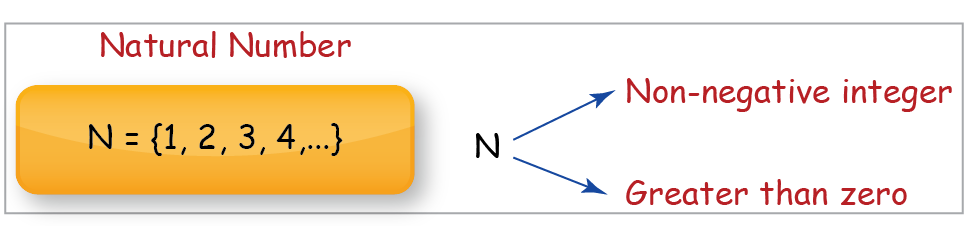We will learn about the various properties of natural numbers as part of understanding this concept.

## Whole Numbers

The whole number does not contain any decimal or fractional part. It means that it represents the whole thing without pieces. It is represented by the symbol W.

Example: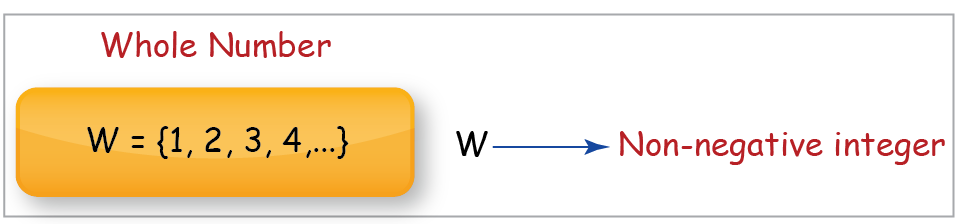We will learn about the various properties of whole numbers as part of understanding this concept.

## Even Numbers

Even numbers are whole numbers that are exactly divisible by $$2.$$

Example: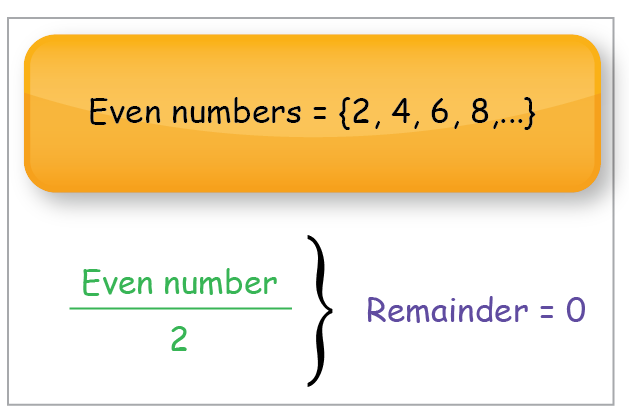## Odd Numbers

Odd numbers are whole numbers that cannot be exactly divided by $$2.$$

Example: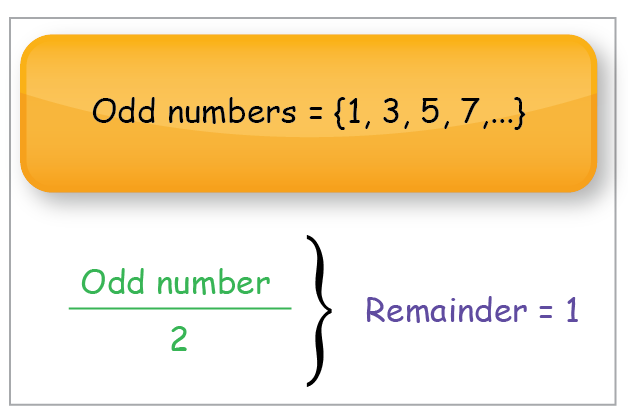## Prime Numbers

Any whole number greater than $$1$$ that has exactly two factors, $$1$$ and itself is defined to be a prime number.

Example: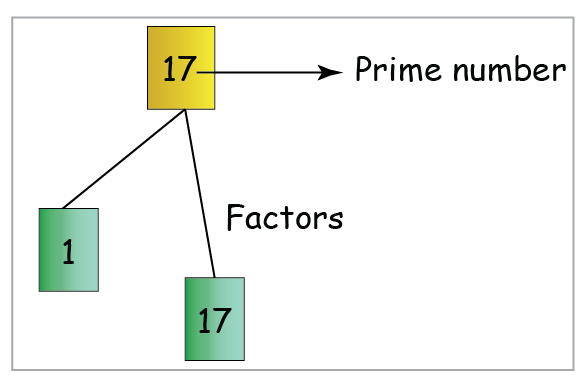Well, now we know that a prime number has just two factors. One and the number itself. But how do we know that a number is prime? You will learn How to find out if a Number is a Prime Number in this section. along with the concepts of Prime Numbers and Euclid’s Proof 3.

## Composite Numbers

Any number greater than $$1$$ that is not a Prime number, is defined to be a composite number. Thus composite numbers will always have more than $$2$$ factors.

Example: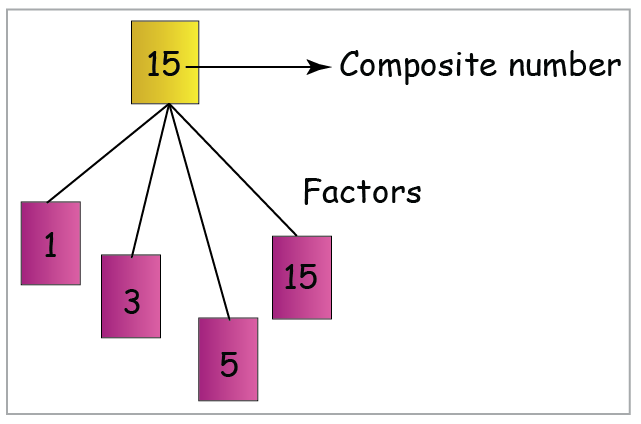Composite numbers are positive integers and you already know that they have more than one factor. Let us now learn about the Fundamental Theorem of Arithmetic

## Co-prime Numbers

If a pair of numbers has no common factor apart from 1, then they are called co-prime numbers.

Example: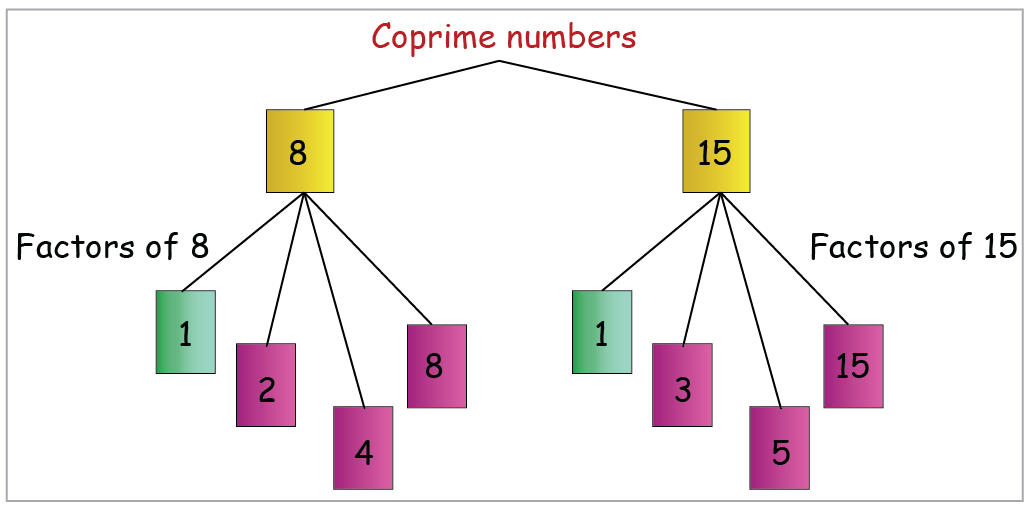## Perfect Numbers

A perfect number is a positive integer that is equal to the sum of its proper divisors (factors), excluding the number itself.

Example: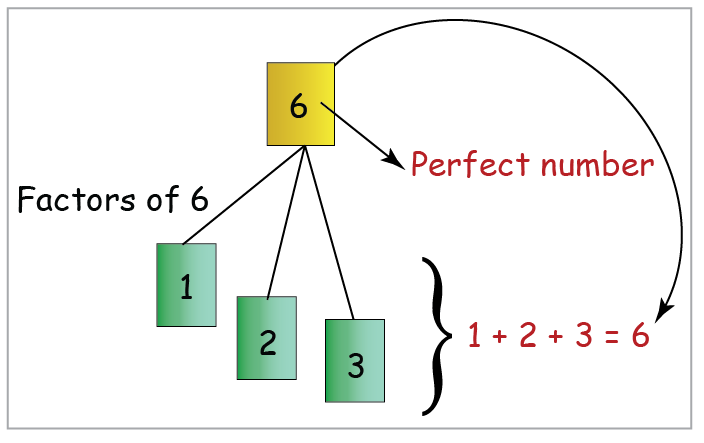## Fractions

Fractions are a part of a whole. They are represented by numbers that have two parts to them. There is a number at the top, which is called the numerator, and the number at the bottom is called the denominator.

Example: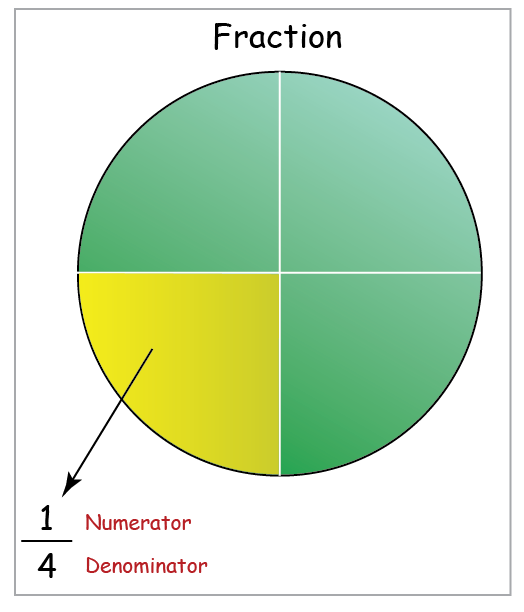Now that we already know about fractions and how it is represented, let us explore some more fraction related topics like Equivalent Fractions, Improper and Mixed Fractions, Addition and Subtraction of Fractions, Multiplication of Fractions and Division of Fractions.

## Decimals

A decimal number has a whole number part and a fractional part. These parts are separated by a decimal point.

Example: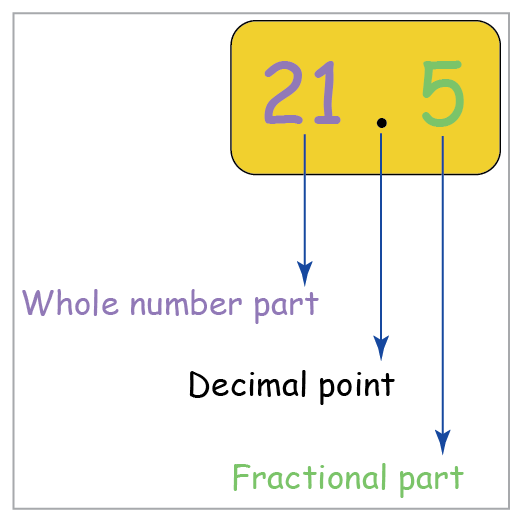Decimals are really interesting. They have a whole number part and they can also be represented as fractions. Let’s dive deeper and find out how! In this section, we will cover decimals related concepts such as the Relationship between Fractions and Decimals, Addition and Subtraction of Decimals, Multiplication of Decimals, and Division of Decimals.

## Rational Numbers

A rational number, denoted by Q, is represented in the form p/q,  where q is not equal to zero.

Integers, Fractions, Decimals, Whole numbers, and Natural numbers are all Rational numbers.

Example: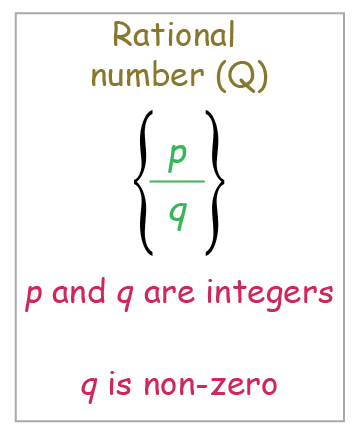In order to get a better understanding of Rational numbers, we will cover topics like Decimal Representation of Rational Numbers, The Rational Line has Irrational Holes, and Operations on Rational Numbers

## Irrational Numbers

Irrational Numbers are the numbers that cannot be represented using integers in the $$\frac{p}{q}$$  form. The set of irrational numbers is denoted by I.

Example: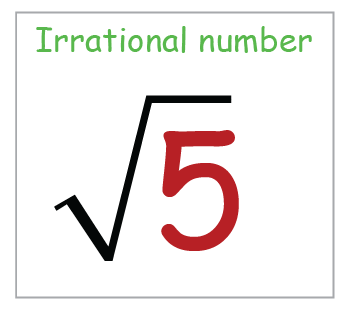Irrational numbers cannot be represented as a simple fraction. Their decimal expansion neither terminates nor becomes periodic. You must be wondering how! We will find out once we study some more topics related to irrational numbers such as Square Root of Two is Irrational, Decimal Representation of Irrational Numbers, The exactness of Decimal Representation, Rationalize the Denominator, Surds, and Conjugates and Rationalization.

## Real Numbers

The set of real numbers is the union of the set of Rationals (Q) and Irrationals (I). It is denoted by R.

Example: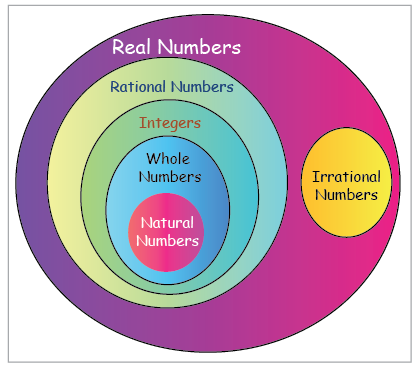## Complex Numbers

A complex number is a number that can be expressed in the form $$a + bi,$$ where $$a$$ and $$b$$ are real numbers, and $$i$$ is a solution of the equation $$x^2 = −1.$$ Because no real number satisfies this equation, $$i$$ is called an imaginary number.

Example: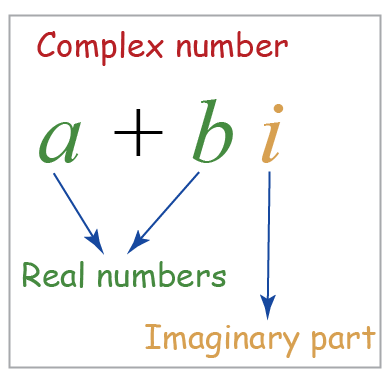Complex numbers have a real part and an imaginary part. Wait, do you think Complex numbers are really complex? Well, let us study them in detail to find out. In this section, we cover different topics like Complex Numbers – Points in the Plane, A Complex Number as a Point in the Plane, What is i? Magnitude and Argument, Powers of iota, Addition and Subtraction of Complex Numbers, Multiplication of Complex Numbers, Conjugate of a Complex Number, Division of Complex Numbers, Addition, Subtraction, and Interpretation of |z1-z2|.

# Factors and Multiples

## Factors

Factors of a given number are numbers that can perfectly divide that given number.

Example: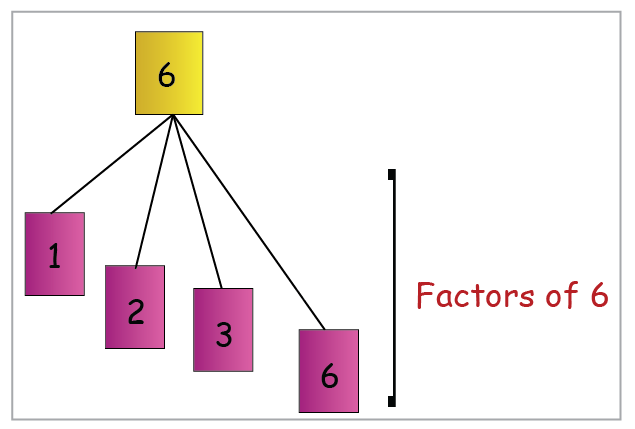## Multiples

A multiple of a number is a number obtained by multiplying the given number by another whole number.

Example: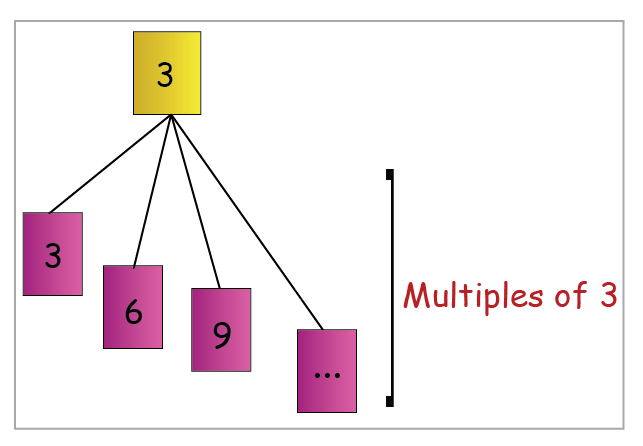## Highest Common Factor (HCF)

The highest common factor (HCF) of two numbers is the largest whole number which is a factor of both. It is also called the Greatest Common Factor (GCF).

Example: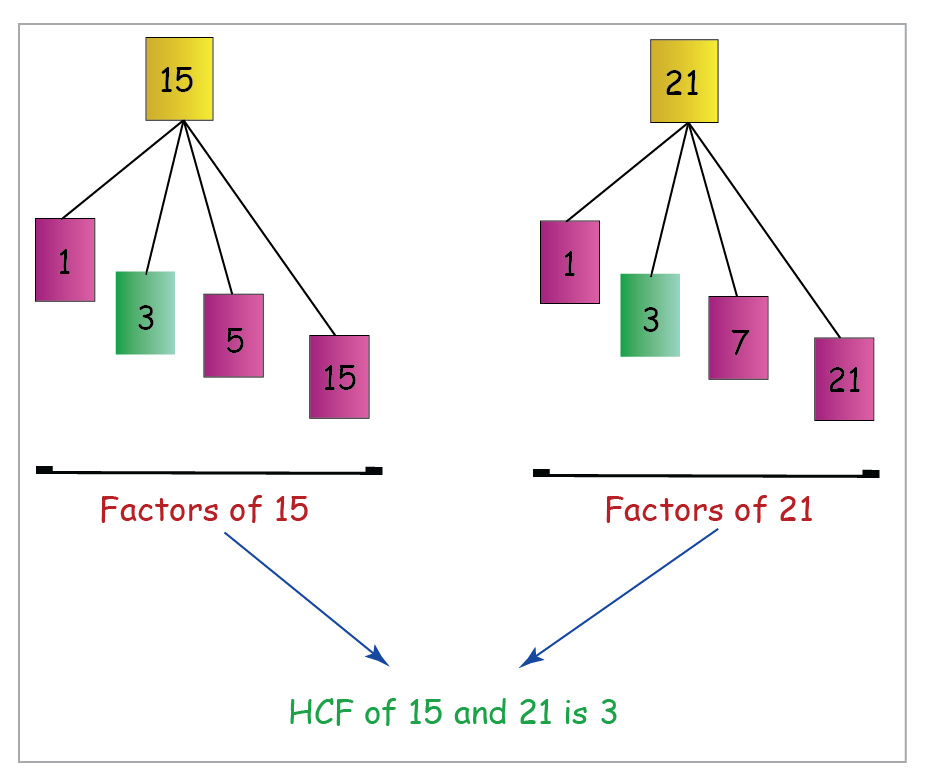## Least Common Multiple (LCM)

When we consider two numbers, each will have its own set of multiples. Some multiples will be common to both numbers.

The smallest of these common multiples is called the least common multiple (LCM) of the two numbers.

Example: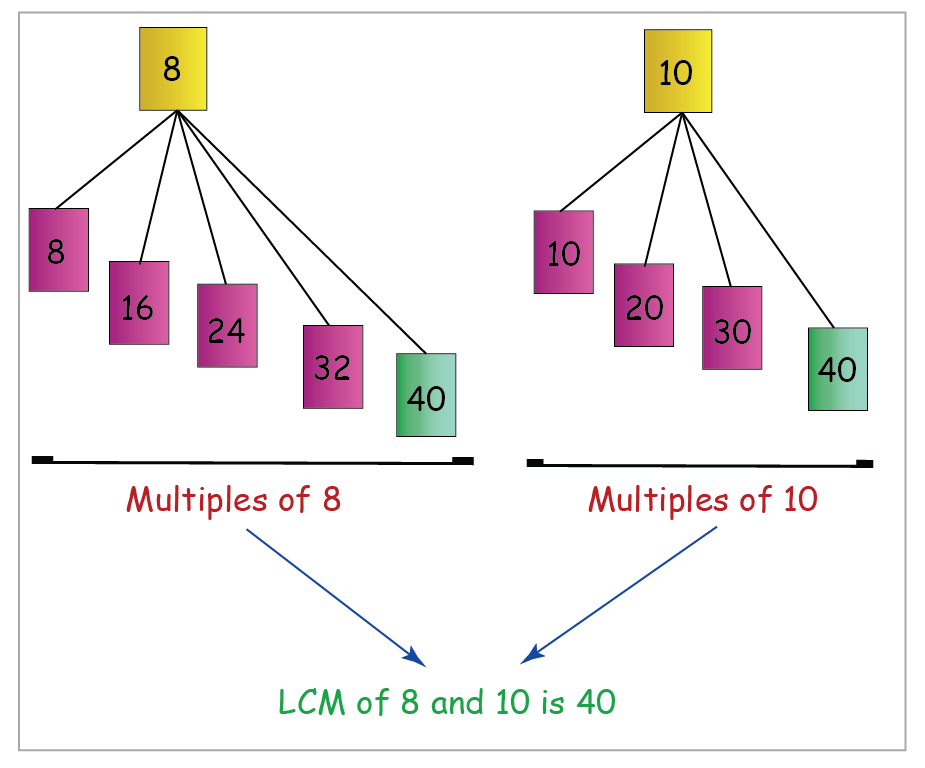## Prime Factorization

Prime factorization allows us to write any number as a product of prime factors.

Example: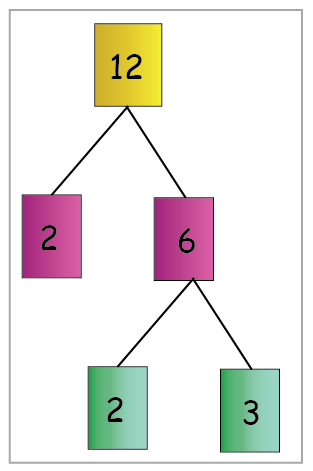To do prime factorization, you need to break a number down to its prime factors. In this section, we will learn about concepts such as Divisibility, GCD, and LCM. We will also have a look at the various Applications of Prime Factorization.